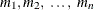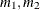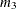### PARMS Statement

`PARMS` (value-list)…</ options> ;

The PARMS statement specifies initial values for the semivariance parameters of a single specified model in the MODEL statement. Alternatively, the PARMS statement can request a grid search over several values of these parameters. You must specify the values by starting with the nugget effect parameter. You continue in the order in which semivariogram forms are specified in the FORM= option of the MODEL statement by specifying for each structure the values for its scale, range, and any other parameters as applicable.

The PARMS statement is optional and must follow the associated MODEL statement.

The value-list specification can take any of several forms:

m

a single valueseveral values

m to n

a sequence in which m equals the starting value, n equals the ending value, and the increment equals 1

m to n by i

a sequence in which m equals the starting value, n equals the ending value, and the increment equals itomixed values and sequences

You can use the PARMS statement to input fixed values for parameters and also initial values that you want to optimize.

Suppose that you want to fit a semivariogram model with a Matérn component of scale 3, range 20, smoothing parameter 4.5, and an exponential component of unspecified scale and range 15. Assume that you also want to fix all the specified parameter values for the optimization. Including the nugget effect, you have a model with six parameters.

In terms of the PARMS statement, your specifications mean that you have initial values for the second, third, fourth, and sixth parameter in the parameter list. Also, the same specifications imply that you provide no initial values for the first parameter (which corresponds to the nugget effect) and the fifth parameter (which corresponds to the exponential model scale). For these parameters you prefer that PROC VARIOGRAM selects initial values, instead. Since you must specify values for all model parameters in the PARMS statement, you simply specify missing values for the first and fifth parameter. This is the way to request that PROC VARIOGRAM assigns default initial values to parameters. The SAS statements to implement these specifications are as follows:

```proc variogram data=FirstData;
< other VARIOGRAM statements >
model form=(mat,exp);
parms (.) (3) (20) (4.5) (.) (15) / hold=(2 to 4,6);
run;
```

Note: The preceding statements are equivalent to the following ones in which the PARMS statement is omitted:

```proc variogram data=FirstData;
< other VARIOGRAM statements >
model form=(mat,exp) scale=(3,.) range(20,15) smooth=4.5;
run;
```

This example might suggest that you can always use either the PARMS or the MODEL statement to specify the same fitting parameters in the VARIOGRAM procedure. However, the PARMS statement gives you more flexibility in two ways:

• You can set non-default initial parameter values by using the PARMS statement, whereas in the MODEL statement you can request default initial values only by setting parameters to missing values. For this reason the PARMS statement cannot be specified when the FORM=AUTO option is specified in the associated MODEL statement. As an example, the following statements do not have an equivalent without using the PARMS statement, because the first parameter in the PARMS statement list (which corresponds to the NUGGET parameter) is set to the specific initial value of 2.1 and the fifth parameter (which corresponds to the exponential structure scale) is set to the specific initial value of 0.3.

```proc variogram data=FirstData;
< other VARIOGRAM statements >
model form=(mat,exp);
parms (2.1) (3) (20) (4.5) (0.3) (15) / hold=(2 to 4,6);
run;
```
• In the MODEL statement all the nonmissing parameter values that you specify remain fixed. Instead, the PARMS statement considers all values in the specified parameter sets to be subjected to optimization unless you force values to be fixed with the HOLD= option. In the previous example, you can specify that you want to optimize all of your parameters by skipping the HOLD= option as shown in the following modified statements:

```proc variogram data=FirstData;
< other VARIOGRAM statements >
model form=(mat,exp);
parms (2.1) (3) (20) (4.5) (1) (15);
run;
```

When you omit the PARMS statement list and the PDATA= data set in a PARMS statement, the specification is equivalent to a PARMS statement list where all the parameters have missing initial values. However, if you specify no other option in the PARMS statement, then the PARMS statement is ignored.

In order to avoid ambiguity, you cannot specify the PARMS statement if any of the scale, range, nugget, or smoothness parameters has been specified in the associated MODEL statement either explicitly or in the MDATA= data set. This condition is in effect even when you specify an empty PARMS statement.

If you specify more than one set of initial values, a grid of initial values sets is created. PROC VARIOGRAM seeks among the specified sets for the one that gives the lowest objective function value. Then, the procedure uses the initial values in the selected set for the fitting optimization.

The results from the PARMS statement are the values of the parameters on the specified grid. The ODS name of the Parameter Search table is ParmSearch.

You can specify the following options after a slash (/) in the PARMS statement:

HOLD=value-list
EQCONS=value-list

specifies which parameter values be constrained to equal the specified values. For example, the following statement constrains the first and third semivariance parameters to equal 0.5 and 12, respectively. The fourth parameter is fixed to the default initial value that is assigned to it by PROC VARIOGRAM.

```parms (0.5) (3) (12) (.) / hold=1,3,4;
```

The HOLD= option accepts only nonmissing values in its list. If you specify more than the available parameters in the HOLD= option list, then the ones in excess are ignored. If the HOLD= option list has integer values that do not correspond to variables in the PARMS list, then they are also ignored. Noninteger values are rounded to the closest integer and evaluated accordingly.

When you specify more than one set of parameter initial values, the HOLD= option list applies to the set that gives the lowest objective function value before this set is sent to the optimizer for the fitting.

LOWERB=value-list

specifies lower boundary constraints on the semivariance parameters. The value-list specification is a list of numbers or missing values (.) separated by commas. You must list the numbers in the order that PROC VARIOGRAM uses for the semivariance parameters, and each number corresponds to the lower boundary constraint. A missing value instructs PROC VARIOGRAM to use its default constraint.

If you do not specify lower bounds for all of the semivariance parameters, then PROC VARIOGRAM assumes that the remaining parameters are not bounded. If you specify more lower bounds in the value-list than the available parameters, then the numbers in excess are ignored. If you specify lower bounds for parameters with missing initial values, then the VARIOGRAM procedure enforces the specified bounds in the fitting process. By default, the lower bound for all parameters is zero.

When you specify the HOLD= option together with the LOWERB= option, the lower bounds in the LOWERB= option value-list that correspond to fixed parameters are ignored. When you specify the NOBOUND option together with the LOWERB= option, the LOWERB= option is ignored.

MAXSCALE=maxscale

specifies a positive upper threshold for the fitted semivariogram sill. This option imposes a linear constraint on the optimization of the nonfixed semivariogram scale and nugget parameters so that the sum of all scale and nugget parameters does not exceed the specified MAXSCALE= value. The MAXSCALE= constraint is ignored if all the semivariogram scale and nugget parameters are fixed.

NOBOUND

requests the removal of boundary constraints on semivariance parameters. For example, semivariance parameters have a default zero lower boundary constraint since they have a physical meaning only for positive values. The NOBOUND option enables the fitting process to derive negative estimates; hence, you need to be cautious with the outcome when you specify this option.

The NOBOUND option has no effect on the power model exponent parameter. The exponent must range within [0,2) so that the model is a valid semivariance function. Also, the NOBOUND option has no effect on the Matérn smoothness parameter. The options LOWERB= and UPPERB= are ignored if either of them is specified together with the NOBOUND option in the PARMS statement.

PARMSDATA=SAS-data-set
PDATA=SAS-data-set

specifies that semivariance parameters values be read from a SAS data set. The data set should contain the values in the sequence required by the PARMS statement in either of the following two ways:

• Specify one single column under the variable `Estimate` (or `Est`) that contains all the parameter values.

• Use one column for each parameter, and place the n columns under the `Parm1``Parm`n variables.

For example, the following two data sets are valid and equivalent ways to specify initial values for the nugget effect and the parameters of the Matérn and exponential structures that have been used in the previous examples in the PARMS statement section:

```data parData1;
input Estimate @@;
datalines;
. 3 20 4.5 . 15
;
```
```data parData2;
input Parm1 Parm2 Parm3 Parm4 Parm5 Parm6;
datalines;
. 3 20 4.5 . 15
;
```

If you have the `parData1` data set, then you can import this information into the PARMS statement as follows:

```proc variogram data=FirstData;
< other VARIOGRAM statements >
model form=(mat,exp);
parms / pdata=parData1 hold=(2 to 4,6);
run;
```

You can specify more than one set of initial values in the PDATA= data set by following the preceding guidelines. PROC VARIOGRAM seeks among the specified sets for the one that gives the lowest objective function value. Then, the procedure uses the initial values in the selected set for the fitting optimization.

You can explicitly specify initial parameter values in the PARMS statement or use the PDATA= option, but you cannot use both at the same time.

UPPERB=value-list

specifies upper boundary constraints on the semivariance parameters. The value-list specification is a list of numbers or missing values (.) separated by commas. You must list the numbers in the order that PROC VARIOGRAM uses for the semivariance parameters, and each number corresponds to the upper boundary constraint. A missing value instructs PROC VARIOGRAM to use its default constraint.

If you do not specify upper bounds for all of the semivariance parameters, then PROC VARIOGRAM assumes that the remaining parameters are not bounded. If you specify more upper bounds in the value-list than the available parameters, then the numbers in excess are ignored. If you specify upper bounds for parameters with missing initial values, then the VARIOGRAM procedure enforces the specified bounds in the fitting process. By default, the scale, range, nugget, and Matérn smoothness parameters have no upper bounds, whereas the power model exponent parameter is lower than two.

When you specify the HOLD= option together with the UPPERB= option, the upper bounds in the UPPERB= option value-list that correspond to fixed parameters are ignored. When you specify the NOBOUND option together with the UPPERB= option, the UPPERB= option is ignored.